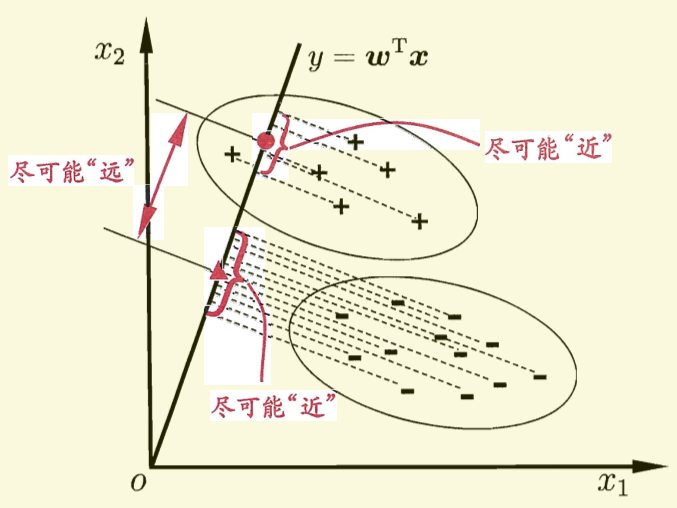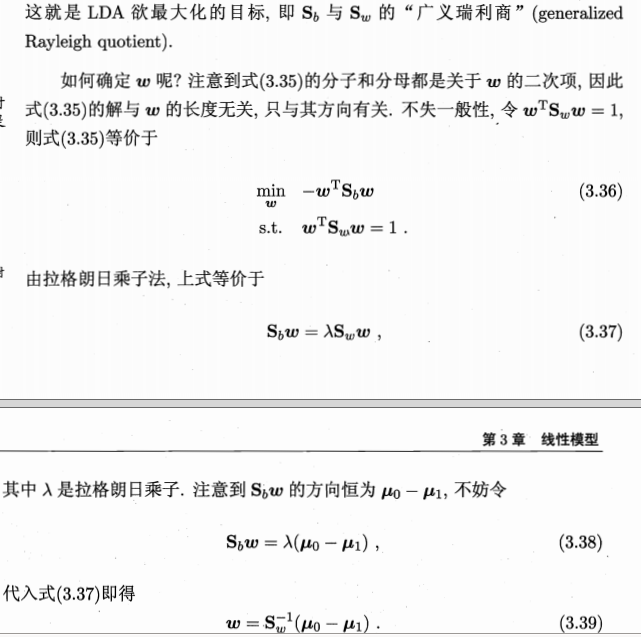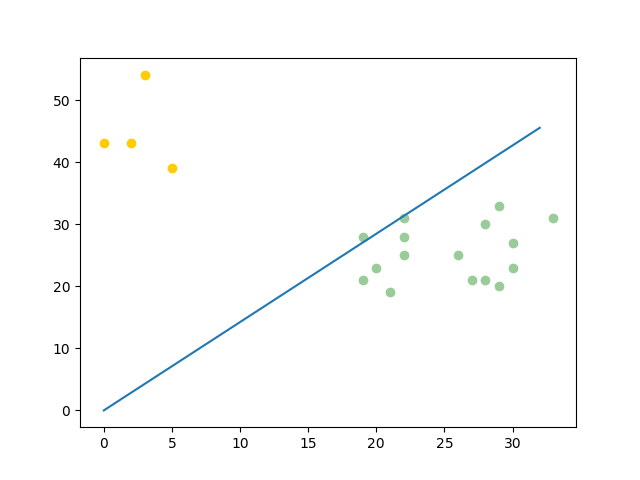# fisher判别分析原理+python实现

## 公式推导ps: 图中有一处描述似乎不是特别的准确，直线的方程应该是

0=wTx

y=wTx

ps: 因为在书关于此的其他讨论中，并未涉及任何y的概念，这里将y写入对我造成了某种误导。

J=wTu0wTu1wT0w+wT0w

Sw=0+1=xX0(xu0)(xu0)T+xX1(xu1)(xu1)T

Sb=(u0u1)(u0u1)T

J可重写为：
J=wTSbwwTSww

ps:sorry 这些公式确实敲得有点累，道个歉，我直接截图了。希望不影响大家的理解。## 编程实现

### 数据生成

from sklearn.datasets import make_multilabel_classification
import numpy as np

x, y = make_multilabel_classification(n_samples=20, n_features=2,
n_labels=1, n_classes=1,
random_state=2)  # 设置随机数种子，保证每次产生相同的数据。

# 根据类别分个类
index1 = np.array([index for (index, value) in enumerate(y) if value == 0])  # 获取类别1的indexs
index2 = np.array([index for (index, value) in enumerate(y) if value == 1])  # 获取类别2的indexs

c_1 = x[index1]   # 类别1的所有数据(x1, x2) in X_1
c_2 = x[index2]  # 类别2的所有数据(x1, x2) in X_2

### fisher算法实现


def cal_cov_and_avg(samples):
"""
给定一个类别的数据，计算协方差矩阵和平均向量
:param samples:
:return:
"""
u1 = np.mean(samples, axis=0)
cov_m = np.zeros((samples.shape, samples.shape))
for s in samples:
t = s - u1
cov_m += t * t.reshape(2, 1)
return cov_m, u1

def fisher(c_1, c_2):
"""
fisher算法实现(请参考上面推导出来的公式，那个才是精华部分)
:param c_1:
:param c_2:
:return:
"""
cov_1, u1 = cal_cov_and_avg(c_1)
cov_2, u2 = cal_cov_and_avg(c_2)
s_w = cov_1 + cov_2
u, s, v = np.linalg.svd(s_w)  # 奇异值分解
s_w_inv = np.dot(np.dot(v.T, np.linalg.inv(np.diag(s))), u.T)
return np.dot(s_w_inv, u1 - u2)

### 判定类别

def judge(sample, w, c_1, c_2):
"""
true 属于1
false 属于2
:param sample:
:param w:
:param center_1:
:param center_2:
:return:
"""
u1 = np.mean(c_1, axis=0)
u2 = np.mean(c_2, axis=0)
center_1 = np.dot(w.T, u1)
center_2 = np.dot(w.T, u2)
pos = np.dot(w.T, sample)
return abs(pos - center_1) < abs(pos - center_2)

w = fisher(c_1, c_2)  # 调用函数，得到参数w
out = judge(c_1, w, c_1, c_2)   # 判断所属的类别
print(out)

### 绘图

import matplotlib.pyplot as plt

plt.scatter(c_1[:, 0], c_1[:, 1], c='#99CC99')
plt.scatter(c_2[:, 0], c_2[:, 1], c='#FFCC00')
line_x = np.arange(min(np.min(c_1[:, 0]), np.min(c_2[:, 0])),
max(np.max(c_1[:, 0]), np.max(c_2[:, 0])),
step=1)

line_y = - (w * line_x) / w
plt.plot(line_x, line_y)
plt.show()• python3
• ubuntu 16.04
• pycharm

02-041万+

#### 主成分分析（PCA）和线性判别分析（LDA）原理简介04-125809

#### Fisher准则线性分类器的Python实现

05-05197

#### python代码完成Fisher判别

05-05291

#### Fisher判别的推导概念和过程+python代码实现（三分类）

01-182万+

#### 判别函数（三）之Fisher线性判别

02-249145

#### 轻松理解————Fisher判别

05-272932

#### ML笔记：LDA(Fisher) 线性判别分析最全解读+python实战二分类代码+补充：矩阵求导可以参考！

05-296509

#### Fisher线性判别分析©️2020 CSDN 皮肤主题: 大白 设计师: CSDN官方博客点击重新获取扫码支付1.余额是钱包充值的虚拟货币，按照1:1的比例进行支付金额的抵扣。
2.余额无法直接购买下载，可以购买VIP、C币套餐、付费专栏及课程。余额充值